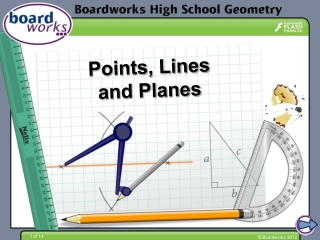DownloadDownload PresentationPoints, Lines and Planes

# Points, Lines and Planes

Télécharger la présentation## Points, Lines and Planes

- - - - - - - - - - - - - - - - - - - - - - - - - - - E N D - - - - - - - - - - - - - - - - - - - - - - - - - - -
##### Presentation Transcript

1. Points, Lines and Planes

2. Information

3. Points, lines and planes

4. Points and lines Apoint is a location in space. It is zero dimensional, meaning it has no height, width or length. A point is represented by a dot and named with a capital letter. A line is set of points that follow a straight path infinitely in either direction. It has only one dimension, which means it has length, but no width or height. A line is represented by a straight line with an arrowhead at either end. A B h Lines can be named two ways: • with a lower case letter h • which can also be written AB.

5. Intersecting lines What do lines m and n have in common? m A The lines share point A. n More mathematically, lines m and nintersect at point A. If two lines intersect, they intersect at exactly one point.

6. Collinear lines What do the points A, B and C have in common? A, B and C all lie on the same line – they are collinear. Is it possible to have two points that are noncollinear? No, a line can always be drawn connecting two points. Through any two points, there is exactly one line.

7. Lines in the coordinate plane Lines can be graphed on a coordinate plane and the intersection defined by giving its coordinates. What is the intersection of the functions f(x)= –2x – 3 and g(x)= x? set equations equal to each other: x =–2x – 3 3x = –3 solve for x: g(x)= x x = –1 f(x) = y use x to find y: f(x) = –2(–1) – 3 f(x) = –1 They intersect at the point (–1, –1). f(x)= –2x – 3 All the points of a linear function are collinear.

8. Rays A ray is a part of a line starting at a point, called the endpoint and extending infinitely in one direction. R S A ray is named using two points and the ray symbol: the endpoint first and any other point along that ray, e.g., RS. Opposite rays share an endpoint but extend in opposite directions so that they form a line. Y X Z Ray XYand XZ form a line and they are opposite rays.

9. Planes Aplane is a flat surface. It has two dimensions: length and width, but no height. A plane extends infinitely in all directions. However, it is usually represented by a parallelogram. B ℛ A C A plane is named with a capital script letter, or with any three noncollinear points that are on it, e.g., ℛ, or plane ABC.

10. Lines on a coordinate plane Why must three noncollinear points by given in order to define a plane? If only two points were given, it would define a line, which could lie in infinitely many planes. Giving a third noncollinear point uniquely defines the plane. A C B

11. Coplanar Geometrical objects are coplanar if they lie in the same plane. Is it possible to have two lines that are noncoplanar? Yes. Two nonintersecting lines. For example, one on a piece of paper and one going through the piece of paper, but not through the line.

12. Lines and planes If two planes intersect, they intersect at exactly one line. A ℋ Points A, B and C define plane ℛ. D ℒ A E Points A, B and D define plane ℋ. C Planes ℛ and ℋ intersect at AB. ℛ B F What points define plane ℒ? Define the intersections of planes ℋ, ℛ, and ℒ. ℒ is defined by any three of the points: C, D, E, and F. ℒ and ℋ intersect at DF. ℒ and ℛ intersect at CE. They all intersect at the origin.

13. True or false?

14. Modeling with geometry